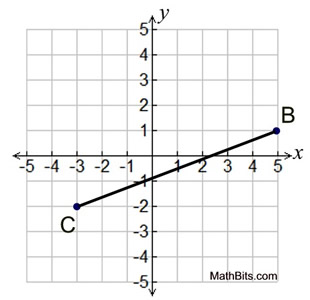Practice - Grid Rotations Terms of Use    Contact Person: Donna RobertsDirections: Read carefully. The point O represents the origin.

1.
a) Find the image of the point (-3,3) after a rotation of 90º counterclockwise about the origin.
Choose:
 (3,3) (-3,-3) (3.-3) (3,-3)

b)
Find the image of the point (-3,3) after a rotation of 270º clockwise about the origin.
Choose:
 (3,3) (-3,-3) (3,-3) (3,-3)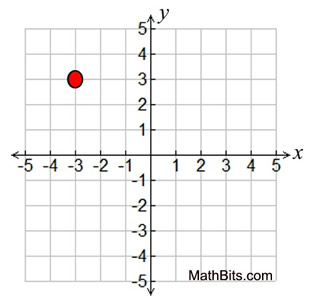2.
ΔABC is rotated 90º counterclockwise about the origin. What are the coordinates of the vertices of its image?
R
O,90º(ΔABC) = (ΔA'B'C')

Choose:
 A' (1,1), B' (-2,-3), C' (-2,1) A' (-1,1), B' (3,-2), C' (1,2) A' (1,-1), B' (-3,2), C' (1,2) A' (1,-1), B' (-3,-2), C' (-1,2)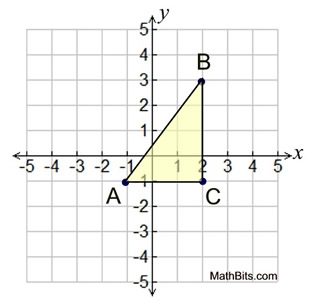3.
What is the image of the point (-5,2) under a rotation of 180º about the origin?

Choose:
 (-5,-2) (5,2) (2,-5) (5,-2)4.
Which of the triangles shown is not a rotation of triangle A about the origin?

Choose:
 B C D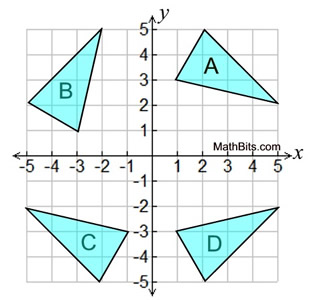5.
A rotation about the origin moves A(3,5) to B(-5,3). What are the coordinates of the image of point (-3,-5) under this same rotation?
Choose:
 (5,-3) (-5,3) (-5,-3) (3,5)6.
Rectangle ABCD has been rotated counterclockwise 180º about the origin, followed by a reflection in the x-axis.
Which of the following single transformations will accomplish this same move?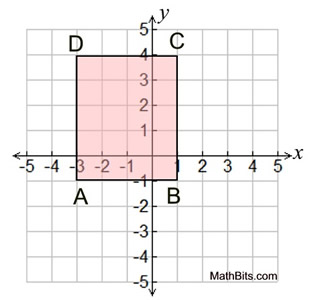Choose:
 90º clockwise rotation in (0,0) reflection over y-axis reflection over line y = x 180º clockwise rotation in (0,0)

7.
ΔFUN is shown at the right. Find the coordinates of vertex U under a clockwise rotation of 90º about the origin.

Choose:
 (3,-4) (-4,3) (4,-3) (-3,-4)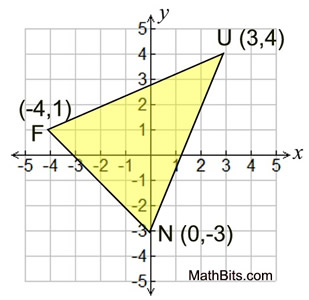8.
Point A is rotated 180º counterclockwise about the origin. If the coordinates of A are (-1,3), what are the coordinates of A', its image?
Choose:
 (-1,-3) (1,-3) (-3,-1) (3,-1)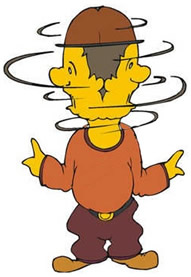9.
In the graph at the right, ΔABC has been transformed into ΔA'B'C'. Which transformation occurred?
(O = origin)

Choose: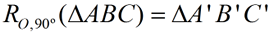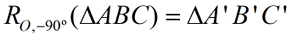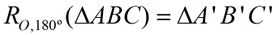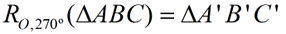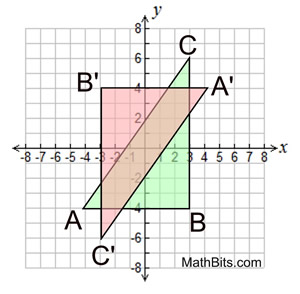10.
The coordinates of the endpoints of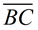are B(5,1) and C(-3,-2), Under the transformation RO, 90º , the image ofis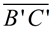. State the coordinates of points B' and C' ?

Choose:
 B' (-1,5), C' (2,3) B' (-5,-1), C' (3,2) B' (-1,-5), C' (2,3) B' (-1,5), C' (2,-3)